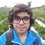# Pre-RMO 2014/15

Let $$XOY$$ be a triangle with $$\angle XOY = 90^\circ$$. Let $$M$$ and $$N$$ be the midpoints of legs $$OX$$ and $$OY$$, respectively. Suppose that $$XN = 19$$ and $$YM = 22$$. What is $$XY$$?

This note is part of the set Pre-RMO 2014Note by Pranshu Gaba
5 years, 9 months ago

This discussion board is a place to discuss our Daily Challenges and the math and science related to those challenges. Explanations are more than just a solution — they should explain the steps and thinking strategies that you used to obtain the solution. Comments should further the discussion of math and science.

When posting on Brilliant:

• Use the emojis to react to an explanation, whether you're congratulating a job well done , or just really confused .
• Ask specific questions about the challenge or the steps in somebody's explanation. Well-posed questions can add a lot to the discussion, but posting "I don't understand!" doesn't help anyone.
• Try to contribute something new to the discussion, whether it is an extension, generalization or other idea related to the challenge.

MarkdownAppears as
*italics* or _italics_ italics
**bold** or __bold__ bold
- bulleted- list
• bulleted
• list
1. numbered2. list
1. numbered
2. list
Note: you must add a full line of space before and after lists for them to show up correctly
paragraph 1paragraph 2

paragraph 1

paragraph 2

[example link](https://brilliant.org)example link
> This is a quote
This is a quote
    # I indented these lines
# 4 spaces, and now they show
# up as a code block.

print "hello world"
# I indented these lines
# 4 spaces, and now they show
# up as a code block.

print "hello world"
MathAppears as
Remember to wrap math in $$ ... $$ or $ ... $ to ensure proper formatting.
2 \times 3 $2 \times 3$
2^{34} $2^{34}$
a_{i-1} $a_{i-1}$
\frac{2}{3} $\frac{2}{3}$
\sqrt{2} $\sqrt{2}$
\sum_{i=1}^3 $\sum_{i=1}^3$
\sin \theta $\sin \theta$
\boxed{123} $\boxed{123}$

Sort by:

Length of XY is 26

- 5 years, 8 months ago

XY = 26

- 5 years, 8 months ago

XY=26

- 5 years, 8 months ago

26

- 5 years, 8 months ago

26

- 5 years, 8 months ago

Let $XM=MO=a$ and $ON=NY=b$

By applying PT, we get

$4a^{2}+b^{2}=19^{2} \rightarrow Eq.1$

$a^{2}+4b^{2}=22^{2} \rightarrow Eq.2$

Eq.1+Eq.2

$a^{2}+b^{2}=\frac{19^{2}+22^{2}}{5}=13^{2}$

$\Rightarrow \sqrt{4a^{2}+4b^{2}}=XY=\sqrt{4× 169}=\boxed{26}$

- 5 years, 9 months ago

is XY 26????

- 5 years, 9 months ago

Yes .XY=26 is the correct answer.

- 1 year, 11 months ago